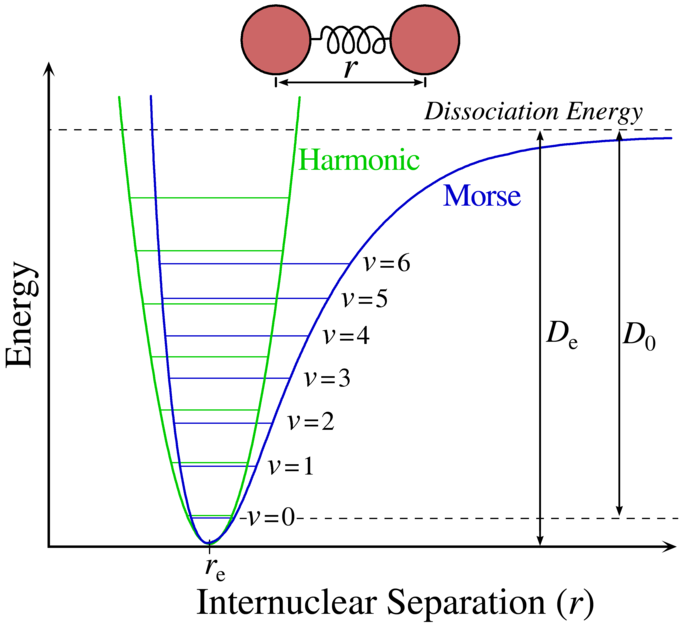## Bond Energy

#### Learning Objective

• Identify the relationship between bond energy and strength of chemical bonds

#### Key Points

• Values listed in tables of bond energy and bond length are averages taken over a variety of compounds that contain a specific atom pair.
• A plot of the potential energy of a two-atom system and the distance between the atoms reveals a distance at which the energy is at its minimum. This distance is the bond length between the atoms.
• The higher the bond energy associated with a specific atom pair, the stronger the bond is said to be, and the smaller the distance between the two atoms.

#### Terms

• Bond EnergyA measure of a chemical bond’s strength. It is experimentally determined by measuring the heat (or enthalpy) required to break a mole of molecules into their constituent individual atoms.
• enthalpyIn thermodynamics, a measure of the heat content of a chemical or physical system, measured under conditions of constant pressure.
• Morse curveA plot showing the dependence of the energy associated with a system of two atoms on the distance between them (referred to as the ‘internuclear distance’).
• equilibrium bond lengthThe average distance between two atoms when they are bonded to each other.

## The Energy Associated with a Chemical Bond

Bond energy is a measure of a chemical bond’s strength, meaning that it tells us how likely a pair of atoms is to remain bonded in the presence of energy perturbations. Alternatively, it can be thought of as a measure of the stability gained when two atoms bond to each other, as opposed to their free or unbound states.

Bond energy is determined by measuring the heat required to break one mole of molecules into their individual atoms, and it represents the average energy associated with breaking the individual bonds of a molecule. The higher the bond energy, the ‘stronger’ we say the bond is between the two atoms, and the distance between them (bond length) is smaller.

For instance, the HO-H bond in a water molecule requires 493 kJ/mol to break and generate the hydroxide ion (OH). Breaking the O-H bond in the hydroxide ion requires an additional 424 kJ/mol. Therefore, the bond energy of the covalent O-H bonds in water is reported to be the average of the two values, or 458.9 kJ/mol. These energy values (493 and 424 kJ/mol) required to break successive O-H bonds in the water molecule are called ‘bond dissociation energies,’ and they are different from the bond energy. The bond energy is the average of the bond dissociation energies in a molecule.

The exact properties of a specific kind of bond are determined in part by the nature of the other bonds in the molecule; for example, the energy and length of the C–H bond will vary depending on what other atoms are bonded to the carbon atom. Similarly, the C-H bond length can vary by as much as 4% between different molecules. For this reason, the values listed in tables of bond energy and bond length are usually averages taken over a variety of compounds that contain a specific atom pair.

We can apply bond energy values to determine the enthalpy of a compound’s formation, [latex]\Delta H_f[/latex], which can be roughly approximated by simply adding tabulated values for the bond energies of all created bonds. The accuracy of this method is within a few percent of the experimentally determined [latex]\Delta H_f[/latex] values.

## Energy Between Two Atoms as a Function of Internuclear Distance

A Morse curve shows how the energy of a two atom system changes as a function of internuclear distance.Morse curvePlot of potential energy vs distance between two atoms. The bond energy is energy that must be added from the minimum of the ‘potential energy well’ to the point of zero energy, which represents the two atoms being infinitely far apart, or, practically speaking, not bonded to each other.

At large distances, the energy is zero, meaning that there is no interaction. This agrees with our understanding that two atoms placed infinitely far apart do not interact with each other in any meaningful way, or at the very least we can say that they are not bonded to each other. At internuclear distances in the order of an atomic diameter, attractive forces dominate. At very small distances between the two atoms, the force is repulsive and the energy of the two atom system is very high. The attractive and repulsive forces are balanced at the minimum point in the plot of a Morse curve.

The internuclear distance at which the energy minimum occurs defines the equilibrium bond length. This bond length represents an ‘equilibrium’ value because thermal motion causes the two atoms to vibrate about this distance, much like a spring vibrates back and forth around its unstretched, or equilibrium distance.

A Morse curve will have different energy minima and distance dependence for bonds formed between different pairs of atoms. In general, the stronger the bond between two atoms, the lower the energy minimum is and the smaller the bond length.

The bond energy is the amount of work that must be done to pull two atoms completely apart; in other words, it is the same as the depth of the “well” in the potential energy curve.